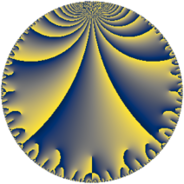Properties

 Label 14.7.dLevel $14$ Weight $7$ Character orbit 14.d Rep. character $\chi_{14}(3,\cdot)$ Character field $\Q(\zeta_{6})$ Dimension $8$ Newform subspaces $1$ Sturm bound $14$ Trace bound $0$

Related objects

Defining parameters

 Level: $$N$$ $$=$$ $$14 = 2 \cdot 7$$ Weight: $$k$$ $$=$$ $$7$$ Character orbit: $$[\chi]$$ $$=$$ 14.d (of order $$6$$ and degree $$2$$) Character conductor: $$\operatorname{cond}(\chi)$$ $$=$$ $$7$$ Character field: $$\Q(\zeta_{6})$$ Newform subspaces: $$1$$ Sturm bound: $$14$$ Trace bound: $$0$$

Dimensions

The following table gives the dimensions of various subspaces of $$M_{7}(14, [\chi])$$.

Total New Old
Modular forms 28 8 20
Cusp forms 20 8 12
Eisenstein series 8 0 8

Trace form

 $$8 q - 128 q^{4} - 336 q^{5} + 652 q^{7} + 756 q^{9} + O(q^{10})$$ $$8 q - 128 q^{4} - 336 q^{5} + 652 q^{7} + 756 q^{9} - 2016 q^{10} - 1356 q^{11} + 2064 q^{14} + 27144 q^{15} - 4096 q^{16} - 17304 q^{17} - 6816 q^{18} - 32004 q^{19} + 9756 q^{21} + 25248 q^{22} - 4128 q^{23} + 10752 q^{24} + 4664 q^{25} - 4704 q^{26} - 7552 q^{28} - 30312 q^{29} + 9648 q^{30} - 3108 q^{31} + 3276 q^{33} + 98028 q^{35} - 48384 q^{36} - 6124 q^{37} + 155568 q^{38} + 100764 q^{39} + 64512 q^{40} - 315936 q^{42} - 297376 q^{43} - 43392 q^{44} - 172116 q^{45} - 194064 q^{46} + 313908 q^{47} + 32432 q^{49} + 38784 q^{50} + 253692 q^{51} + 255360 q^{52} + 278484 q^{53} + 386064 q^{54} - 125952 q^{56} - 81288 q^{57} - 169824 q^{58} - 835464 q^{59} - 434304 q^{60} - 995316 q^{61} + 1216188 q^{63} + 262144 q^{64} + 8316 q^{65} + 1673280 q^{66} + 648808 q^{67} + 553728 q^{68} - 1572816 q^{70} + 190128 q^{71} - 218112 q^{72} - 1617084 q^{73} - 1158144 q^{74} - 2042208 q^{75} + 1456224 q^{77} + 1122432 q^{78} + 70096 q^{79} + 344064 q^{80} + 1177920 q^{81} + 66528 q^{82} - 1133568 q^{84} + 2190984 q^{85} - 573024 q^{86} - 2057076 q^{87} - 403968 q^{88} + 739116 q^{89} + 2233752 q^{91} + 264192 q^{92} - 23364 q^{93} + 3795120 q^{94} + 725640 q^{95} - 344064 q^{96} - 3532320 q^{98} - 4625928 q^{99} + O(q^{100})$$

Decomposition of $$S_{7}^{\mathrm{new}}(14, [\chi])$$ into newform subspaces

Label Dim. $$A$$ Field CM Traces $q$-expansion
$a_{2}$ $a_{3}$ $a_{5}$ $a_{7}$
14.7.d.a $8$ $3.221$ $$\mathbb{Q}[x]/(x^{8} - \cdots)$$ None $$0$$ $$0$$ $$-336$$ $$652$$ $$q+(\beta _{2}-\beta _{3})q^{2}+(-\beta _{2}+2\beta _{3}-\beta _{6}+\cdots)q^{3}+\cdots$$

Decomposition of $$S_{7}^{\mathrm{old}}(14, [\chi])$$ into lower level spaces

$$S_{7}^{\mathrm{old}}(14, [\chi]) \cong$$ $$S_{7}^{\mathrm{new}}(7, [\chi])$$$$^{\oplus 2}$$